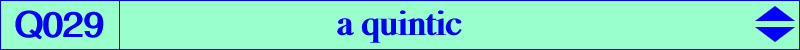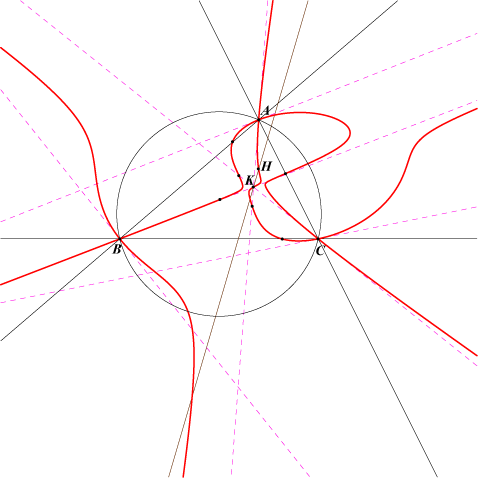too complicated to be written here. Click on the link to download a text file.X(4), X(6), X(54), X(895)Consider the triangle formed by the reflections of P in the vertices of ABC and the circumanticevian triangle of P. These two triangles are perspective if and only if P lies on Q029 or on the line at infinity. Q029 is a quintic with double points A, B, C. The tangents at A are the lines through K and the point 0 : b^2(b^2 - 3c^2) : c^2(3b^2 - c^2). The fifth point on BC is 0 : a^2 - 3b^2 : a^2 - 3c^2 and the fifth point on AK is a^2 : 2b^2 : 2c^2.